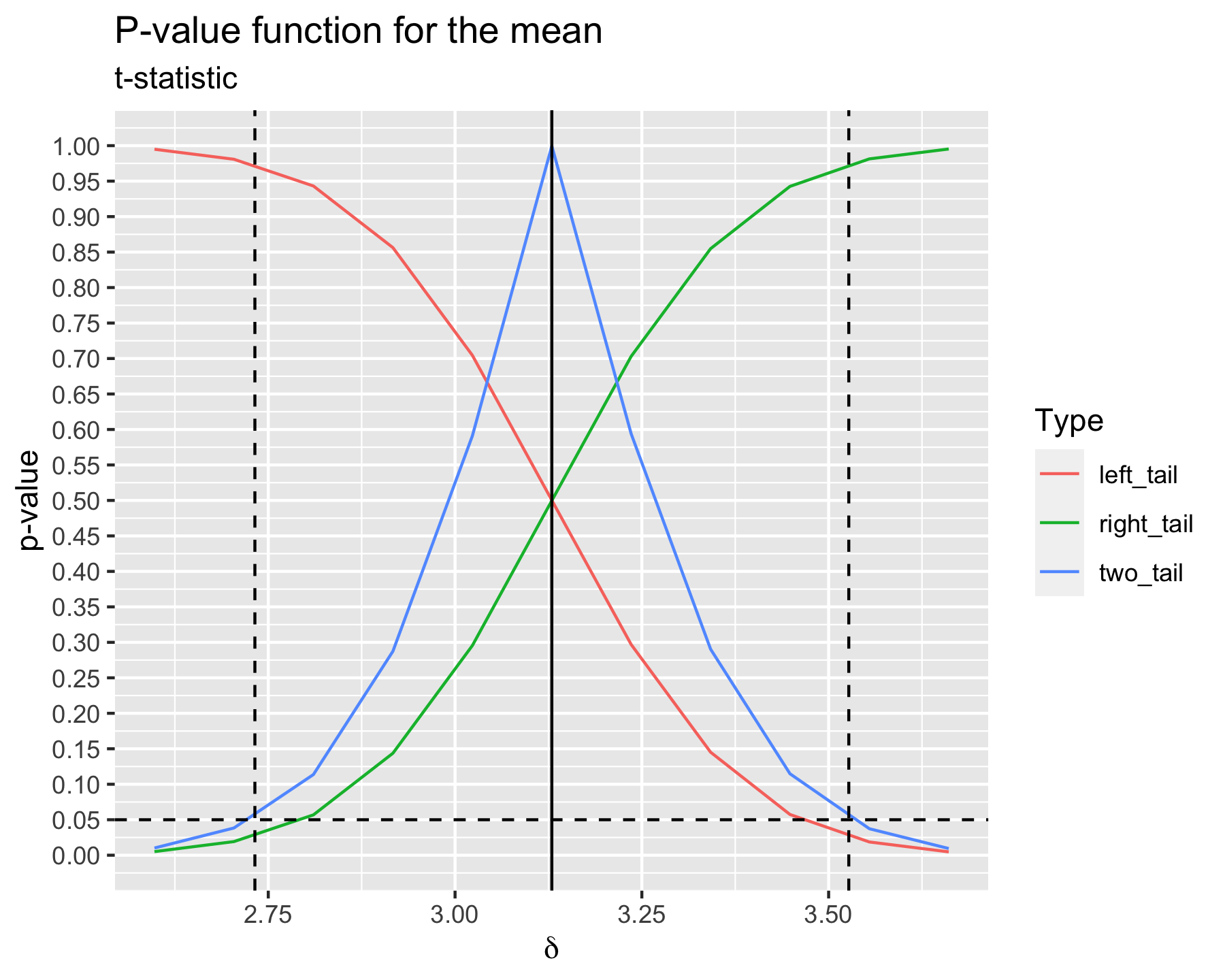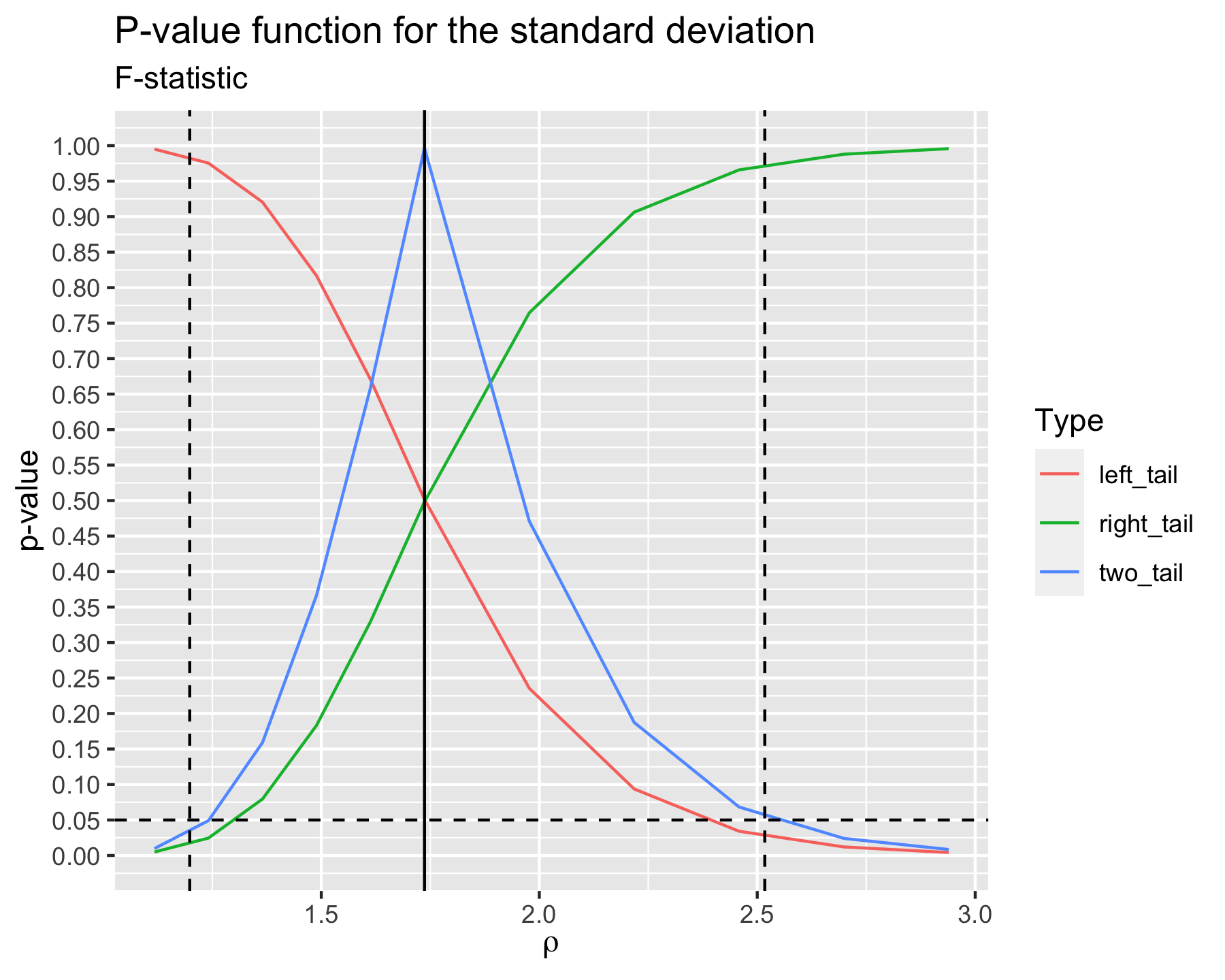# Computing plausibility functions

ngrid_in <- 10
ngrid_out <- 100
nperms <- 100000
n1 <- 30
n2 <- 30
set.seed(1301)
x1 <- rnorm(n1, mean = 0, sd = 1)
x2 <- rnorm(n2, mean = 3, sd = 1)
y1 <- rnorm(n1, mean = 0, sd = 1)
y2 <- rnorm(n2, mean = 0, sd = 2)
z1 <- rnorm(n1, mean = 0, sd = 1)
z2 <- rnorm(n2, mean = 3, sd = 2)

The concept of plausibility functions pertains to assessing the $$p$$-value of a set of null hypotheses and to plot this $$p$$-value surface on the domain defined by the set of null hypotheses. The idea behind is that, if such a plausibility function is available, you can deduce from it point estimates or confidence interval estimates for parameters used to define the nulls or extract a single $$p$$-value for a specific null of interest (Martin 2017; Fraser 2019; Infanger and Schmidt-Trucksäss 2019). In particular, there is another R package dedicated to plausibility functions called pvaluefunctions.

## Plausibility function for the mean

null_spec <- function(y, parameters) {
map(y, ~ .x - parameters)
}
stat_functions <- list(stat_t)
stat_assignments <- list(delta = 1)

pf <- PlausibilityFunction$new( null_spec = null_spec, stat_functions = stat_functions, stat_assignments = stat_assignments, x1, x2, seed = 1234 ) pf$set_nperms(nperms)
pf$set_point_estimate(mean(x2) - mean(x1)) pf$set_parameter_bounds(
point_estimate = pf$point_estimate, conf_level = pf$max_conf_level
)
pf$set_grid( parameters = pf$parameters,
npoints = ngrid_in
)

pf$set_alternative("two_tail") pf$evaluate_grid(
grid = pf$grid, ncores = 1 ) df <- rename(pf$grid, two_tail = pvalue)

pf$set_alternative("left_tail") pf$grid$pvalue <- NULL pf$evaluate_grid(
grid = pf$grid, ncores = 1 ) df <- bind_rows( df, rename(pf$grid, left_tail = pvalue)
)

pf$set_alternative("right_tail") pf$grid$pvalue <- NULL pf$evaluate_grid(
grid = pf$grid, ncores = 1 ) df <- bind_rows( df, rename(pf$grid, right_tail = pvalue)
)

pf$set_grid( parameters = pf$parameters,
npoints = ngrid_out
)

df_mean <- tibble(
delta = pf$grid$delta,
two_tail = approx(df$delta, df$two_tail, delta)$y, left_tail = approx(df$delta, df$left_tail, delta)$y,
right_tail = approx(df$delta, df$right_tail, delta)$y, ) %>% pivot_longer(-delta) df_mean %>% ggplot(aes(delta, value, color = name)) + geom_line() + labs( title = "P-value function for the mean", subtitle = "t-statistic", x = expression(delta), y = "p-value", color = "Type" ) + geom_hline( yintercept = 0.05, color = "black", linetype = "dashed" ) + geom_vline( xintercept = mean(x2) - mean(x1), color = "black" ) + geom_vline( xintercept = stats::t.test(x2, x1, var.equal = TRUE)$conf.int,
color = "black",
linetype = "dashed"
) +
scale_y_continuous(breaks = seq(0, 1, by = 0.05), limits = c(0, 1))## Plausibility function for the variance

null_spec <- function(y, parameters) {
map(y, ~ .x / parameters)
}
stat_functions <- list(stat_f)
stat_assignments <- list(rho = 1)

pf <- PlausibilityFunction$new( null_spec = null_spec, stat_functions = stat_functions, stat_assignments = stat_assignments, y1, y2, seed = 1234 ) pf$set_nperms(nperms)
pf$set_point_estimate(sd(y2) / sd(y1)) pf$set_parameter_bounds(
point_estimate = pf$point_estimate, conf_level = pf$max_conf_level
)
pf$set_grid( parameters = pf$parameters,
npoints = ngrid_in
)

pf$set_alternative("two_tail") pf$evaluate_grid(
grid = pf$grid, ncores = 1 ) df <- rename(pf$grid, two_tail = pvalue)

pf$set_alternative("left_tail") pf$grid$pvalue <- NULL pf$evaluate_grid(
grid = pf$grid, ncores = 1 ) df <- bind_rows( df, rename(pf$grid, left_tail = pvalue)
)

pf$set_alternative("right_tail") pf$grid$pvalue <- NULL pf$evaluate_grid(
grid = pf$grid, ncores = 1 ) df <- bind_rows( df, rename(pf$grid, right_tail = pvalue)
)

pf$set_grid( parameters = pf$parameters,
npoints = ngrid_out
)

df_sd <- tibble(
rho = pf$grid$rho,
two_tail = approx(df$rho, df$two_tail, rho)$y, left_tail = approx(df$rho, df$left_tail, rho)$y,
right_tail = approx(df$rho, df$right_tail, rho)$y, ) %>% pivot_longer(-rho) df_sd %>% ggplot(aes(rho, value, color = name)) + geom_line() + labs( title = "P-value function for the standard deviation", subtitle = "F-statistic", x = expression(rho), y = "p-value", color = "Type" ) + geom_hline( yintercept = 0.05, color = "black", linetype = "dashed" ) + geom_vline( xintercept = sqrt(stats::var.test(y2, y1)$statistic),
color = "black"
) +
geom_vline(
xintercept = sqrt(stats::var.test(y2, y1)$conf.int), color = "black", linetype = "dashed" ) + scale_y_continuous(breaks = seq(0, 1, by = 0.05), limits = c(0, 1))## Plausibility function for both mean and variance Assume that we have two r.v. $$X$$ and $$Y$$ that differ in distribution only in their first two moments. Let $$\mu_X$$ and $$\mu_Y$$ be the means of $$X$$ and $$Y$$ respectively and $$\sigma_X$$ and $$\sigma_Y$$ be the standard deviations. We can therefore write $Y = \delta + \rho X.$ In this case, we have $\begin{cases} \mu_Y = \delta + \rho \mu_X \\ \sigma_Y^2 = \rho^2 \sigma_X^2 \end{cases} \Longleftrightarrow \begin{cases} \delta = \mu_Y - \frac{\sigma_Y}{\sigma_X} \mu_X \\ \rho = \frac{\sigma_Y}{\sigma_X} \end{cases}$ In the following example, we have $$\delta = 3$$ and $$\rho = 2$$. null_spec <- function(y, parameters) { map(y, ~ (.x - parameters) / parameters) } stat_functions <- list(stat_t, stat_f) stat_assignments <- list(delta = 1, rho = 2) pf <- PlausibilityFunction$new(
null_spec = null_spec,
stat_functions = stat_functions,
stat_assignments = stat_assignments,
z1, z2,
seed = 1234
)
pf$set_nperms(nperms) pf$set_point_estimate(c(
mean(z2) - sd(z2) / sd(z1) * mean(z1),
sd(z2) / sd(z1)
))
pf$set_parameter_bounds( point_estimate = pf$point_estimate,
conf_level = pf$max_conf_level ) # Fisher combining function pf$set_aggregator("fisher")
pf$set_grid( parameters = pf$parameters,
npoints = ngrid_in
)
pf$evaluate_grid(grid = pf$grid, ncores = 1)
grid_in <- pf$grid pf$set_grid(
parameters = pf$parameters, npoints = ngrid_out ) if (requireNamespace("interp", quietly = TRUE)) { Zout <- interp::interp( x = grid_in$delta,
y = grid_in$log_rho, z = grid_in$pvalue,
xo = sort(unique(pf$grid$delta)),
yo = sort(unique(pf$grid$log_rho))
)
pf$grid$pvalue <- as.numeric(Zout$z) } else pf$grid$pvalue <- rep(NA, nrow(pf$grid))

df_fisher <- pf$grid # Tippett combining function pf$set_aggregator("tippett")
pf$set_grid( parameters = pf$parameters,
npoints = ngrid_in
)
pf$evaluate_grid(grid = pf$grid, ncores = 1)
grid_in <- pf$grid pf$set_grid(
parameters = pf$parameters, npoints = ngrid_out ) if (requireNamespace("interp", quietly = TRUE)) { Zout <- interp::interp( x = grid_in$delta,
y = grid_in$log_rho, z = grid_in$pvalue,
xo = sort(unique(pf$grid$delta)),
yo = sort(unique(pf$grid$log_rho))
)
pf$grid$pvalue <- as.numeric(Zout$z) } else pf$grid$pvalue <- rep(NA, nrow(pf$grid))

df_tippett <- pf\$grid
df_fisher %>%
ggplot(aes(delta, log_rho, z = pvalue)) +
geom_contour_filled(binwidth = 0.05) +
labs(
title = "Contour plot of the p-value surface",
subtitle = "Using Fisher's non-parametric combination",
x = expression(delta),
y = expression(log(rho)),
fill = "p-value"
) +
theme_minimal()